# JP2005045723A - Image correcting apparatus - Google Patents

## Info

Publication number
JP2005045723A
JP2005045723A JP2003280156A JP2003280156A JP2005045723A JP 2005045723 A JP2005045723 A JP 2005045723A JP 2003280156 A JP2003280156 A JP 2003280156A JP 2003280156 A JP2003280156 A JP 2003280156A JP 2005045723 A JP2005045723 A JP 2005045723A
Authority
JP
Japan
Prior art keywords
image
paper
contour
mesh
nodes
Prior art date
Legal status (The legal status is an assumption and is not a legal conclusion. Google has not performed a legal analysis and makes no representation as to the accuracy of the status listed.)
Granted
Application number
JP2003280156A
Other languages
Japanese (ja)
Other versions
JP4082303B2 (en
Inventor
Eigo Nakagawa
Tetsukazu Satonaga
Koki Uetoko
Kaoru Yasukawa

Original Assignee
Fuji Xerox Co Ltd

Priority date (The priority date is an assumption and is not a legal conclusion. Google has not performed a legal analysis and makes no representation as to the accuracy of the date listed.)
Filing date
Publication date
Application filed by Fuji Xerox Co Ltd, 富士ゼロックス株式会社 filed Critical Fuji Xerox Co Ltd
Priority to JP2003280156A priority Critical patent/JP4082303B2/en
Publication of JP2005045723A publication Critical patent/JP2005045723A/en
Application granted granted Critical
Publication of JP4082303B2 publication Critical patent/JP4082303B2/en
Expired - Fee Related legal-status Critical Current
Anticipated expiration legal-status Critical

• 230000000875 corresponding Effects 0 claims abstract description 25
• 238000003384 imaging method Methods 0 claims abstract description 16
• 238000000034 methods Methods 0 claims description 42
• 238000006243 chemical reaction Methods 0 claims description 38
• 238000003702 image correction Methods 0 claims description 23
• 230000001131 transforming Effects 0 claims description 20
• 238000007689 inspection Methods 0 claims description 10

## Images

•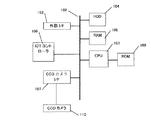•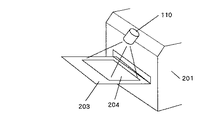•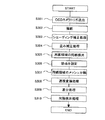•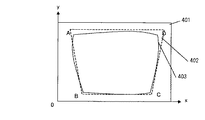•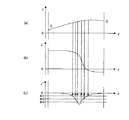•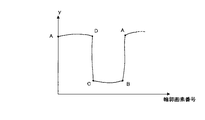•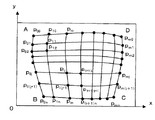•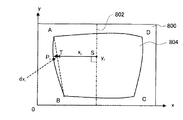•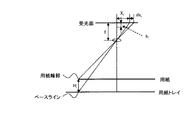•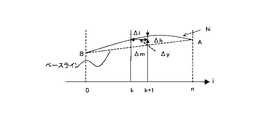•••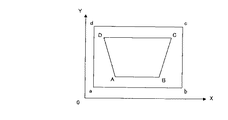•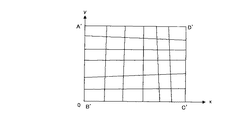•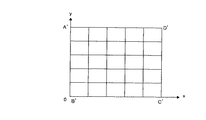## Abstract

<P>PROBLEM TO BE SOLVED: To provide an image correcting apparatus which accurately converts an image resulting from imaging a warped or distorted original into an image in the state of flattening a paper sheet. <P>SOLUTION: An image area ABCD of a paper sheet portion is extracted from the image resulting from imaging the warped paper sheet or the like, nodal points P<SB>00</SB>-P<SB>mn</SB>are set onto sides AB, BC, CD, DA of the image area ABCD so as to be tight as a curve of each side is sharpened. The nodal points on the confronted sides (AD and BC, for example,) are made correspondent in order from the right side and from the upside, and dividing lines connecting these corresponding nodal points are drawn to divide the image area ABCD into meshes. At such a time, the dividing line connecting the nodal point pairs on the sides AD and BC is approximately shaped into the curve of the side AB as the pair is close to the side AB or approximately shaped into the curve of the side DC as the pair is close to the side DC. The rectangular meshes resulting from the division are perspective-transformed and assembled into a rectangular mesh for a target rectangular area, thereby constructing the image in the state of flattening the paper sheet. <P>COPYRIGHT: (C)2005,JPO&NCIPI

## Description

The present invention relates to a technique for correcting a captured image obtained by imaging a sheet having warpage or deflection into a state without warping or deflection.

Conventionally, as an apparatus for reading an image, there are an apparatus for reading an image by directly contacting an original like a flat bed scanner or a sheet scanner, and an apparatus for reading an image non-contact by an area sensor or the like. In the former type, the image is read with the document pressed against the platen glass or line sensor. Therefore, the document can always be read with the document facing the image sensor. There is a problem that the reading speed is slow because of the large scan or scanning. On the other hand, since the latter type uses a two-dimensional area sensor, it is not necessary to perform scanning for reading. Therefore, there is a merit that a reduction in size and speed can be achieved and a reading apparatus can be realized at a low price.

As an example of such a non-contact type image reading apparatus, Patent Document 1 discloses an apparatus having a function of reading a document from an oblique upper side using an area sensor and converting the read image into an image taken from the front. Has been. However, in the case of non-contact scanning, since there is no mechanism for pressing the document against the platen glass or the sensor, unevenness due to warpage or deflection is often generated on the scanned document. For this reason, even if an image read from oblique information is simply perspective-transformed and converted to an image from the front viewpoint, there is a problem that image distortion due to warping or deflection of the paper remains.

To deal with this problem, Japanese Patent Laid-Open No. 2004-228867 extracts a contour from document information read by a camera, extracts vertex information from the contour information, and determines the distance between the camera and the document from the vertex information and known document shape information. An apparatus for measuring and correcting the document information bent from the distance information and vertex information on a plane is disclosed. The apparatus of Patent Document 2 divides a document image into triangular patches by connecting vertices on a side of the document image and vertices on a side facing the document image. Then, the 3D coordinates of each vertex are determined from the vertex information and distance information, and each triangle patch is perspectively transformed on the same plane based on the 3D coordinates of these vertices. Create a blank image.

However, since the technique of Patent Document 2 performs patch division by connecting vertices on two opposite sides of a document image, the document warps or bends along a direction perpendicular to the extending direction of the sides. If this is the case, there is a problem that conversion with high accuracy cannot be performed. In the case of reading a book or notebook that is assumed in Patent Document 2, it may be rare for the paper surface to warp or bend in a direction perpendicular to the spread direction, but in the case of reading a printing result on a sheet of paper. Must be assumed to cause warping and deflection in both the vertical and horizontal directions of the page.

JP 2000-022869 A JP 2002-165083 A

An object of the present invention is to provide an apparatus capable of producing an image when a paper surface to be read is warped in both the vertical and horizontal directions or bent in a horizontal direction, with higher accuracy than before. And

An apparatus according to the present invention is an image correction apparatus that corrects a paper image included in a captured image obtained by imaging a paper in a non-contact manner into an image in a state where the paper is stretched flat. A contour node setting unit that detects a contour line of the paper image from the captured image and sets a plurality of contour nodes on the contour line, and sets a plurality of internal nodes inside the contour line based on the position of each contour node Then, by connecting the contour nodes and the internal nodes with line segments, mesh dividing means for dividing the paper image into a plurality of meshes, and individually converting each mesh divided by the mesh dividing means, Image conversion means for forming a corrected image corresponding to an image in a state where the paper is stretched flat.

In a preferred aspect of the present invention, the contour node setting means sets the same number of contour nodes for every two opposite sides of the four sides constituting the contour line of the paper image, and the mesh dividing means is the paper The interiors that form a quadrilateral with these three nodes based on the positions of the three predetermined contour nodes or internal nodes that are adjacent to form a "<" shape in order from the four corners of the image to the inside. The internal node group is set by repeating the process of determining the position of the node.

In a further preferred aspect, the mesh dividing means determines the positions of the internal nodes in which the three adjacent positions constitute a quadrilateral together with predetermined contour nodes or internal nodes, on four sides of the paper image. Of the vectors formed by connecting adjacent contour nodes, four vectors corresponding to the quadrilateral are used.

In a further preferred aspect, the mesh dividing means performs a weighted average of the two vectors of the two opposite sides of the four vectors according to the distance between the two opposite sides and the quadrilateral. The direction of each of the two vectors extending from the node which is the two end points of the “<” shape to the internal node of the position determination target is obtained from the vector.

In a further preferred aspect, the contour node setting means sets the position of each contour node so that the density of the contour nodes increases for each side constituting the contour of the paper image, as the curve is steeper. Decide.

In another preferred aspect of the present invention, the image converting means meshes a target area to be occupied by an image in which the paper is stretched flat according to a mesh division result of the paper image by the mesh dividing means. Dividing and calculating a formula for perspective transformation between each node that becomes the vertex of the mesh of the target area and each node of the mesh corresponding to the mesh in the paper image, and each mesh of the paper image is calculated by this formula Perform perspective transformation.

In a further preferred aspect, the image conversion means divides each side of the target area in accordance with an interval between adjacent contour nodes on each side of the paper image when meshing the target area. Based on the division result, the target area is divided into meshes.

In another preferred aspect of the present invention, the image processing apparatus includes a target area dividing unit that divides a target area to be occupied by an image in a state where the paper is stretched flat into rectangular mesh groups by vertical and horizontal straight lines, The paper image is mesh-divided according to the result of mesh division by the target area dividing means.

The printing system according to the present invention includes a printing apparatus that prints an input original image on a sheet, an imaging apparatus that captures a printing surface of the sheet printed by the printing apparatus, and an image captured by the imaging apparatus. The image correction apparatus according to any one of claims 1 to 8 is corrected by the image correction apparatus for correcting the sheet image included in the image into an image in a state where the sheet is stretched flat. An inspection device that inspects the quality of printing on the paper by the printing unit by comparing the paper image with the original image.

The best mode for carrying out the present invention will be described with reference to the drawings.

FIG. 1 is a system configuration diagram for explaining an example of a hardware configuration of a print control apparatus to which the present invention is applied. This print control apparatus is an apparatus that controls an IOT (Image Output Terminal) that forms an image on a sheet. The print control apparatus receives print data from a host device such as a PC (personal computer), converts the print data into image data that can be processed by the IOT, supplies the image data to the IOT, and executes image formation. For example, this printing control apparatus is built in the same housing as the IOT to constitute a printing apparatus. A copying machine can be configured by incorporating a reading device for reading an image of a document into the printing device.

A CPU (central processing unit) 101 is a device that executes arithmetic processing for operation control of the printing control apparatus. A ROM (Read Only Memory) 109 stores various programs for operation control of the print control apparatus. In particular, in the context of the present invention, the ROM 109 stores a program for output image inspection processing including read image correction processing described later. The CPU 101 executes a control program in the ROM 109 while using a RAM (Random Access Memory) 105 as a work area. The RAM 105 is used as a work area for general print control processing, a buffer area for sending image data for printing to the IOT via the IOT controller 106, and a work area for output image inspection processing. An HDD (Hard Disk Drive) 104 stores print job data received from the host device, image data for printing that can be developed by expanding the data, and the like. Further, when the printing apparatus is configured as a copying machine, image data obtained by reading a paper document can be stored in the HDD 104 for processing such as sort output. The HDD 104 includes an area for storing image data for printing sent from the outside and an area for storing at least one piece of the image data for inspection of the print result.

The CPU 101 is connected to the data bus 102. Image data is exchanged between each hardware in the print control apparatus through this data bus. In addition to the CPU 101, HDD 104, and RAM 105 described above, an external interface circuit 103, an IOT controller 106, and a CCD camera interface circuit 107 are connected to the data bus 102.

The external interface circuit 103 is a communication interface with the host device. Specific examples of the external interface circuit 103 include a parallel port connected to a printer port of a PC and an Ethernet (trademark) interface.

The IOT controller 106 is an interface circuit between the print control apparatus of FIG. 1 and the IOT. Although not shown, the IOT controller 106 rearranges the image data in a format that can be handled by the IOT in the order of processing, supplies the image data to the IOT, and controls the IOT to execute printing. In the present invention, the type of IOT is not limited, but examples of the IOT include a laser printer and an inkjet printer.

The CCD camera interface circuit 107 converts the output data of the CCD camera 110 from analog to digital and stores it in the RAM 105. The CCD camera 110 is a camera that captures an image of a printed surface of a sheet printed by IOT for inspection of an output image (an image printed on a sheet). The CCD camera 110 includes a two-dimensional CCD area sensor, and the area sensor images a paper print surface without performing mechanical scanning. For example, as shown in FIG. 2, the CCD camera 110 is installed on the upper portion of the paper discharge tray 203 from which the printing result of the printing apparatus 201 is discharged in a positional relationship in which the entire paper discharge tray 203 is placed in the photographing range. The paper discharge tray 203 is formed so that its upper surface has a color different from that of the paper 204. This is a configuration for extracting a printed paper area from an image captured by the CCD camera 110.

FIG. 3 is a flowchart showing a procedure of output image inspection processing by the print control apparatus. This process is executed each time the print control apparatus receives a print job from the outside and executes the print process, and printing of one sheet is completed.

First, when it is detected that the printed paper has been discharged to the paper discharge tray 203, when the CPU 101 issues a shooting start command to the CCD camera interface circuit 107, the interface circuit 107 causes the CCD camera to respond accordingly. A shooting trigger is sent to 110 (S301). In response to this trigger, the CCD camera 110 photographs the upper surface of the newly discharged paper on the paper discharge tray 203 (S302).

A captured image taken by the CCD camera 110 is temporarily stored in the RAM 105. The CPU 101 executing the image inspection processing program reads the captured image from the RAM 105 and executes shading correction (S303). The shading correction is a process for correcting illuminance unevenness in the photographing region, characteristic variation of each pixel of the CCD sensor, and the like. Shading correction is a well-known process. To explain it simply, for example, a blank sheet is photographed in advance to obtain and store a white reference DW [i, j] (i, j is an index representing a two-dimensional pixel position). Then, the value of each pixel of the captured image D [i, j] to be corrected is corrected by the following equation.

P [i, j] = D [i, j] / DW [i, j] × (2 ^ n−1) (1)
n is the bit resolution after correction, and if 8-bit resolution, n = 8 and the pixel value takes a value of 0 to 255 gradations. Here, the method of holding the white reference data for all the pixels has been described. For example, as another simple method, a method of setting the peak value of the entire captured image as the white reference DW, For example, a method using the peak value DW [j] for each line of the image as a white reference can be applied.

Next, the CPU 101 performs optical distortion correction on the captured image subjected to shading correction (S304). Optical distortion correction is a process of correcting the curvature aberration of the optical system of the CCD camera 110. Various known processing methods can be used for optical distortion correction, but an example is as follows. That is, the aberration d at the incident angle θ to the lens is expressed by the following equation, where c is the distance from the lens to the imaging plane and r is the distance from the optical axis of the imaging position on the imaging plane.

d = r−ctanθ (2)
Correction processing is performed based on this characteristic. Or since aberration d is generally proportional to the cube of r, correction processing can be performed by obtaining a proportionality constant based on lens characteristics.

Next, the CPU 101 detects the contour of the printed paper region from the captured image that has been subjected to the optical distortion correction (S305). In the present embodiment, since the color of the upper surface of the paper discharge tray 203 and the paper are different, the color difference is used to specify the area of the printed paper image in the captured image (hereinafter referred to as a paper area image). be able to. A known technique can also be used for this processing. As an example, an edge detection filter is applied to a captured image that has been corrected for optical distortion, and among the edges obtained thereby, the outermost closed edge is used as the paper outline, and the inside is used as the paper region image. There are ways to do it.

FIG. 4 schematically shows an example of a captured image obtained by photographing the printed paper on the paper discharge tray 203. In the apparatus configuration shown in FIG. 2, since the printed paper 204 on the paper discharge tray 203 is photographed obliquely from above with the CCD camera 110, if the printed paper 204 is not warped, bent, bent or the like. The rectangular paper should be deformed into a trapezoidal shape 402 indicated by a broken line 402 with respect to the captured image area 401 on the captured image data. On the other hand, when the printed paper 204 is warped, bent, or the like, the image of the paper 204 in the captured image data is distorted in the outline of the paper as indicated by the solid line 403 and slightly deviated from the trapezoid. Shape. FIG. 4 shows an example of the paper area image when the printed paper is only warped and bent, and there is no bending, and points A, B, C, and D are the apexes at the four corners of the paper. It corresponds to.

When the contour of the printed paper (and the paper region image surrounded thereby) is detected from the captured image in this way, the CPU 101 next places the contour of the paper region image in the captured image on the basis of the contour information. A plurality of nodes are set (that is, the positions of the nodes are determined) (S306). The nodes set on these contours are called contour nodes. Contour nodes are arranged more densely in areas where the side is more bent. As a result, the section between adjacent contour nodes on the side can be regarded as a substantially straight line. A specific example of the contour node setting process will be described in detail later.

Next, the CPU 101 mesh-divides the paper region image with the set contour node group (S307). In this mesh division, as shown in FIG. 7, two sets of opposite sides of the outline are paired in order of the extending direction of the two sides, for example, from the right or from the top, respectively. By connecting the node pairs with lines, the paper region image is mesh-divided into a substantially grid pattern. However, in the paper area image, the edges of the contour are curved due to the warp and deflection of the paper. Therefore, the pair of contour nodes on opposite sides is not connected with a straight line, but another pair of opposite sides is bent. It is connected with a line of the reflected shape (which is conceptually a curve, but is actually a broken line approximating that curve due to the discretization of mesh division). Such a line is called a dividing line. For example, it is assumed that the dividing line connecting the node on the side AD and the node on the side BC reflects the curved shape of the side AB or the side DC. The dividing line connecting each pair of the node of the side AD and the node of the side BC is closer to the curved shape of the side AB as the pair is closer to the side AB, and closer to the curved shape of the side DC as the pair is closer to the side DC. To. In other words, the shape of the dividing line that connects the node pairs gradually changes from the shape of the side AB to the shape of the side DC as the position of the dividing line changes from the side AB to the side DC. To. A specific example of this mesh division processing will be described in detail later.

When mesh division is performed in this way, the CPU 101 next performs perspective transformation for each mesh, and by combining the transformation results, the printed paper with no warpage, deflection, bending, etc. is viewed from the front. The state image is reconstructed (S308). Details of the conversion and image reconstruction processing will be described later.

Next, the CPU 101 reconstructs the paper region image corrected by the above-described processing, that is, the viewpoint, the warp, the deflection, and the like as an image (hereinafter referred to as an original image) indicated by the print data on which the print result is based. By comparing, the quality of the image formed on the printed paper is inspected. The original image to be compared may be stored in the RAM 105 at the time of executing the printing process until the comparison processing stage. As this comparison processing, various known processing methods can be used. Generally speaking, in this comparison process, the difference between the reconstructed paper area image and the original image is calculated (S308), and the image of this difference is examined to detect image defects on the printed paper. (S310). That is, for example, if the print result is dirty, a black dot remains in the difference image obtained by subtracting the original image from the reconstructed paper area image, and conversely, if there is a print defect, the reconstructed paper area image is removed from the original image. Black spots remain in the subtracted difference image. Therefore, it is possible to detect an image defect such as a black spot by binarizing the difference image by setting an appropriate density threshold value. If an image defect is detected, a warning is issued on the user interface, a warning is issued to the host device via the network using print management software, etc., and further via the network via a remote maintenance system, etc. It is also possible to notify the printer maintenance company.

In the above, detection of local image defects such as black spots has been described. However, image quality defects such as overall density unevenness and density shift can also be formed in a pixel area having a value greater than 0 in the above-described difference image. Can be detected.

The overall processing of the apparatus according to the present embodiment has been described above. Next, a specific example of the contour node setting process in S306 will be described.

Here, the nodes are set according to a strategy in which the nodes are set denser as the contour curve is steeper. An example of this setting process will be described with reference to FIG.

FIG. 5 is a diagram showing a concept of setting a node for the side CD in the outline 403 of the paper region image shown in FIG. (A) shows the state of the side CD when the y-coordinate is taken on the horizontal axis and the x-coordinate is taken on the vertical axis. (B) is obtained by linearly differentiating a curve x = c (y) (c () is a function of y) of the side CD shown in the graph of (a) by y. That is, (b) is a graph in which x ′ = dc (y) / dy is plotted. In the calculation process, the first-order differential is obtained by taking the first-order difference of the x coordinate. (C) is obtained by secondarily differentiating the curve x = c (y) of the graph of (a) by y. In the calculation process, the second-order difference is calculated by taking the difference of the x ′ coordinate with respect to the first-order difference (shown in the graph of (b)) of the curve x = c (y), and the second-order difference is calculated. Let x ″.

Since the secondary derivative x ″ obtained in this way indicates the rate of change of the slope of the curve x = c (y), the curve x = c (y) becomes sharper as the secondary derivative x ″ increases. You can say that. Therefore, nodes are set more densely as the secondary differential x ″ is larger.

In the example of FIG. 5, the graph of the second-order difference value x ″ of x in (c) is divided by thresholds th 1 , th 2 ,... At regular intervals, and y at the point where the graph of x ″ intersects with these threshold values. The coordinate value is the y coordinate value of the node. The coordinates (x, y) of each node can be specified by obtaining the x coordinate of the curve x = c (y) corresponding to the y coordinate value of each node from the graph (a). According to such processing, nodes can be set densely as the curve of the contour curve is steeper. However, the method shown here is merely an example, and there are various other methods for setting nodes more densely in a portion where the curve of the contour curve is sharper. Note that the points C and D at both ends of the side CD are also selected as nodes.

Although the method for setting the nodes on the side CD has been described above, the node group can be set similarly for the other sides AB, BC, and DA.

The contour node setting processing described above is processing after the points A, B, C, and D at the four corners of the paper in the paper region image are known. In order to obtain the points A, B, C, and D at the four corners of the paper, for example, the adjacent pixels are sequentially traced on the contour line of the paper region image, and the y and x coordinate values of each contour pixel are plotted in order. Go. FIG. 6 is a graph in which the y-coordinate values of the contour pixels are plotted according to the arrangement order of the contour pixels. In this graph, when the second-order difference for the contour pixel number of the y coordinate is calculated, the second-order difference values appear at points A, B, C, and D where the contour is bent as a value that is significantly larger than the other portions. Therefore, among the peaks of the second-order difference value graph, a peak whose second-order difference value exceeds a predetermined threshold is determined as a peak corresponding to a point at which the contour is bent, and the pixel number and y coordinate of the peak are obtained. The same processing is performed for the x coordinate, and the pixel number and x coordinate of the peak corresponding to the point where the contour is bent are obtained. Then, by combining the x and y coordinates of the peaks having the same pixel number, the coordinates of the point at which the contour is bent can be obtained.

In general, printed sheets discharged from a printing apparatus are rarely bent even if they are warped or bent as shown in the example of FIG. Can be identified. If the contour line can be divided into sides at the four corner points in this way, the coordinate value of each pixel constituting the side is examined for each side, and if either x or y is a variable, that side can be used as a function. Can be expressed. If each side can be expressed as a function in this way, a node can be set on each side by the above-described method.

In the case where the printed paper is bent, a graph of coordinate values along the arrangement of contour pixels as shown in FIG. 6 is obtained in the same manner, and the second-order difference is obtained. Points can be determined. Then, by dividing the outline of the paper region image at each bending point and applying the above-described method for each divided portion, a node can be set. In this case, a bending point is also selected as a node.

However, since the printed paper output from the printing apparatus rarely bends, there is generally no problem if the four corner points of the paper are obtained as described with reference to FIGS.

If the number of nodes on the opposite side is different, the subsequent processing cannot be executed. If the number of nodes on the opposite side is different, for example, the second floor for the side with the smaller number of nodes. By reducing the threshold interval of the difference value, the number of nodes is increased so that the number coincides with the larger number of nodes. Although it is conceivable to match the side having the smaller number of nodes, it is better to match the side having the larger number of nodes in order to improve the conversion accuracy.

Next, a specific example of the mesh division process in S307 will be described with reference to FIG.

In this process, based on the outline node group set on the outline of the paper region image, the positions of the mesh nodes (which are called internal nodes with respect to the outline nodes) are sequentially obtained from the outside to the inside. By connecting these internal node groups with line segments, a dividing line is formed that connects the node pairs on opposite sides (and reflects the curved shape of the other opposite side). Divided into multiple quadrilateral meshes.

A method for determining the position of the internal node will be described with a specific example. Here, the coordinates (x 11 , y 11 ) of the node p 11 in the quadrilateral mesh p 00 p 01 p 11 p 10 (p kl (k, l is an integer of 0 to n) in FIG. 7) are shown. This will be described by taking as an example the case of obtaining. That is, in this example, among the four nodes constituting the quadrilateral mesh, the three points p 00 , p 01 , and p 10 are contour nodes, and their position coordinates are known, whereas the positions of the remaining node p 11 Since the coordinates are unknown, this is calculated.

In this calculation, first, a vector (a, b) parallel to the side p 01 p 11 is obtained. This uses the vector p 00 p 10 = (x 10 −x 00 , y 10 −y 00 ) and the vector p 0n p 1n = (x 1n −x 0n , y 1n −y 0n ), and further the side p 00 From the ratio of the y coordinate components of p 01 and p 01 p 0n (ratio between the lengths in the y direction),

a = {(y 0n −y 01 ) × (x 10 −x 00 ) + (y 01 −y 00 ) × (x 1n −x 0n )} / (y 0n −y 00 ) (3)

b = {(y 0n −y 01 ) × (y 10 −y 00 ) + (y 01 −y 00 ) × (y 1n −y 0n )} / (y 0n −y 00 ) (4)

Is calculated as These equations (3) and (4) are expressed as follows: vectors p 00 p 10 and vectors p 0n and p 1n connecting adjacent contour nodes are represented by vectors (a and p) on the lines connecting the starting points p 00 and p 0n of these vectors. b) a weighted average is calculated by the internal ratio of the position of the start point p 01 of the.

And thus the obtained vector component (a, b) using the coordinates of the vertices p 01, obtains the vertex p 01 the street vectors (a, b) parallel to the linear equation y = f (x).

Similarly, a vector component (c, d) parallel to the side p 10 p 11 is changed into a vector p 00 p 01 = (x 01 −x 00 , y 01 −y 00 ) and a vector p m0 p m1 = (x m1 − x m0 , y m1 −y m0 ) and the ratio of the x coordinate components (length in the x direction) of the sides p 00 p 10 and p 10 p m0 , the same formula as the above formulas (3) and (4) Calculated by Then, using the coordinates of the vector component (c, d) and the vertex p 10 , a linear expression y = g (x) passing through the vertex p 10 and parallel to the vector (c, d) is obtained.

Then, the coordinates of the intersection of y = f (x) and y = g (x) are calculated, and this coordinate is determined as the coordinates (x 11 , y 11 ) of the unknown node p 11 . Since the coordinates need to be obtained in units of pixels, when the intersection coordinates become decimal, they are rounded off and converted to integers.

When the coordinates of the internal node p 11 are obtained in this way, the next is the position coordinates of the nodes p 01 , p 02 , and p 11 , the vector p 01 p 11 , the vector p 0n p 1n , and the vector p 01 p Using the information of 02 and the vector p m1 p m2 , the position of the unknown internal node p 12 can be determined by the same method as described above. In this way, the position of each internal node can be determined in order from the four corners of the substantially trapezoidal paper area image inward.

As described above, in this calculation process, it is assumed that the positions of three of the four nodes constituting the quadrilateral mesh are known, and the positions of the remaining one unknown node are determined based on the three known points. It is obtained from the position information of the nodes and the positions of the contour nodes (total 8) at the positions where the mesh dividing lines including the sides of the quadrilateral mesh intersect with the contour lines of the paper region image. For example, quadrilateral meshes p ij p i (j + 1 ) p (i + 1) (j + 1) p (i + 1) p of the j (i + 1) (j + 1) are the unknown nodal If there is, the remaining three nodes p ij , p i (j + 1) , p (i + 1) j and contour nodes p i0 , p (i + 1) 0 , p in , p (i + 1) ) n, p 0j, p 0 (j + 1), p mj, from p m (j + 1), to calculate the unknown nodal p (i + 1) (j + 1). In other words, the intersection of the strip-shaped region delimited by two adjacent mesh dividing lines in the vertical direction and the strip-shaped region delimited by two adjacent mesh dividing lines in the horizontal direction in the paper region image In the case of the quadrilateral mesh of interest, the unknown nodes of the quadrilateral mesh are the information on the three known nodes of the quadrilateral mesh and the contour nodes at the two ends of the two strip-shaped regions that intersect (four per one strip-shaped region). ), The coordinates of unknown nodes are obtained.

By calculating the coordinates of unknown internal nodes in this way, one quadrilateral mesh is formed. Then, by repeating the process of calculating another unknown node using the calculated internal node as a known node, the entire paper region image can be divided into quadrilateral mesh groups.

When mesh division is completed in this way, each mesh is then subjected to perspective transformation (S308), and the warp and distortion of the paper are corrected and the image of the paper viewed from the front is reconstructed. For this perspective transformation process, a transformation formula (or transformation matrix) is obtained for each mesh. In order to calculate the conversion formula, in the present embodiment, a conversion destination mesh to which each mesh (conversion source) in the paper region image is converted is specified, and the nodes of the conversion source and conversion destination meshes are connected to each other. The conversion formula is calculated from the correspondence. Therefore, first, the conversion destination mesh specifying process will be described.

As one of element processes for specifying the conversion destination mesh, there is a process for obtaining the apparent height h i (i = 0 to n) of the sheet in the captured image. This process will be described with reference to FIGS.

By the method described above, and the i-th node P i when the side AB divided into n constituting the contour of the sheet area image 804, the relationship between the central vertical line 802 of the imaging area 800 of the CCD camera 110 Figure It is shown in FIG. Here, when a perpendicular is drawn from the point P i on the side AB to the vertical line 802, it is assumed that the value of the y coordinate of the foot S of the perpendicular is y i . Further, when the intersection of a base line (indicated by a broken line in the figure) connecting the vertex A and the vertex B with this perpendicular line P i S is T, the length of the line segment TS is assumed to be x i. . Further, the length of the line segment P i T, and the displacement dx i node P i with respect to the base line AB.

Next, in FIG. 9, in a three-dimensional space, when a warped or bent paper is viewed from the y-axis direction (that is, the line-of-sight direction is the y-axis direction), the outline of the paper and the baseline on the image sensor light receiving surface That is, the correspondence relationship on the captured image) is shown. Paper contour is at the height H i to the base line in the real space, forms an image at a distance only dx i than the baseline in the captured image. When the distance from the lens to the light receiving surface is f, the apparent height h i of the paper on the image sensor is

h i = dx i × f / (x i + dx i ) (5)

It becomes. Since dx i , x i and f are known, h i can be calculated. The calculation of the equation (5) is the same processing as that disclosed in Japanese Patent Laid-Open No. 10-65877.

h i indicates the height of a point on the paper outline with respect to the baseline when a three-dimensional xyz coordinate system having the same scale as the xy coordinate system of the captured image is considered. By performing this process on each contour node on each side of the contour of the paper image region, the height of the contour node on each side with respect to the baseline corresponding to that side can be obtained.

When the height of each contour node with respect to the baseline is determined in this way, in the conversion destination mesh specifying process, the height information is then used to use the edges AB, BC, and CD of the paper with warping and deflection. , DA, the length between adjacent nodes on the side when the line is extended straight is obtained. Here, this processing will be described using FIG. 10A and FIG. 10B and taking the side AB as an example.

In this process, an apparent length Δl in the baseline direction of each divided section when the bent side AB is expanded in the baseline direction is calculated. As shown in FIG. 10A, Δl can be approximated as:

Δl = √ (Δy 2 + Δh 2 ) (6)
Here, Δy = y k + 1 −y k (0 ≦ k ≦ n−1) and Δh = h k + 1 −h k (0 ≦ k ≦ n−1). y k and h k are the height of the contour node relative to the y coordinate and the baseline, respectively. When the curled side AB is expanded in the baseline direction based on Δl calculated for each section in this way, the result is as shown in FIG. 10B.

Here, the ratio of Δl calculated for each section is the division of the side corresponding to the side AB in the rectangular region to be obtained after perspective conversion of the paper region image 804 (ie, the rectangle when the paper is viewed from the front). It becomes a ratio.

FIG. 11 shows a rectangular area after the perspective transformation is performed. The vertices A′B′C′D ′ in FIG. 11 sequentially correspond to the vertexes ABCD at the four corners of the paper area image in the captured image before perspective transformation. Here, the side A′B ′ is divided by the ratio of Δl of each divided section described above. Accordingly, the side A′B ′ is divided into the same number of divisions with the same division section length ratio as the side AB. The vertical side A′B ′ has been described above, but the horizontal side A′D ′ can also be divided in the same manner.

Then, by drawing straight lines parallel to the x-axis and y-axis from the dividing points on the sides A′B ′ and A′D ′, the rectangular area is divided into rectangular mesh groups. Each of these rectangular meshes becomes a perspective transformation destination of a quadrilateral mesh at a corresponding position in the paper region image.

A conversion formula for this perspective conversion is calculated for each mesh. In the calculation of the conversion formula, the x- and y-coordinates of the four corners of the conversion destination rectangular mesh and the conversion quadrilateral mesh are substituted into the general formula of the perspective conversion, and the simultaneous equations obtained as a result are solved. be able to. The calculation of the perspective transformation formula will be described below.

Consider a conversion from a rectangular image abcd, which is an image when a rectangular sheet is viewed from the front, to a trapezoidal image ABCD, which is an image when the same rectangular sheet is viewed from an oblique direction, as shown in FIG. At this time, perspective transformation from the point (x, y) in the rectangular image to the point (X, Y) in the trapezoidal image corresponding to the point is, as is well known, the following equations (7) and (8). expressed.

X = (ax + by + c) / (px + qy + 1) (7)
Y = (dx + ey + f) / (px + qy + 1) (8)

Accordingly, there are eight parameters for defining perspective transformation, a to f, and p and q. However, if the coordinates of the four vertices of the rectangular image and the trapezoid image are substituted into the above equations (7) and (8), 8 parameters. Since simultaneous equations consisting of two equations are obtained, the values of eight parameters can be obtained by solving these simultaneous equations. In this way, it is possible to specify a perspective transformation formula for transforming a rectangular paper image viewed from the front into an image viewed obliquely.

The quadrilateral mesh in the captured image can be regarded as a rectangular mesh in the front image of the target paper facing diagonally. Since the coordinates of the vertices of the four corners of each mesh are obtained by the above calculation, by substituting the coordinates of these points into the transformation formulas (7) and (8), the quadrilateral mesh can be obtained from the points on the rectangular mesh. It is possible to calculate unknown parameters a to f and p and q of the conversion formula to the upper point.

By substituting the coordinates (x P , y P ) of an arbitrary pixel P in the rectangular mesh of the front image of the target paper into the equations (7) and (8) into which these parameter values are substituted, The coordinates (X P , Y P ) of the corresponding point of the pixel P in the quadrilateral mesh can be calculated. Therefore, the value of the pixel P in the target rectangular mesh can be obtained from the value of the pixel group in the vicinity of the corresponding point (X P , Y P ) in the quadrilateral mesh. This is, for example, a process such as a weighted average of the values of each pixel in the vicinity of the corresponding point according to the proximity between the corresponding point and the corresponding point, similar to the process used for resolution conversion of the bitmap image. Good.

By executing the above calculation process for each mesh, the paper area image obtained by viewing the paper with warpage and deflection from an angle is corrected to the image viewed from the front with the warpage and deflection flattened. be able to.

By comparing the corrected image with the image indicated by the print data on the sheet (S309 and S310), it is possible to detect defects in the image printed on the sheet.

Although the embodiment of the present invention has been described above, according to this embodiment, even when three-dimensional unevenness such as curling occurs on the output paper, the paper region in the captured image is divided into a mesh shape, By performing a perspective conversion process every time and generating an image viewed from the front, it is possible to perform conversion with higher accuracy, and thus it is possible to inspect for the presence or absence of image defects with higher accuracy.

In the prior art disclosed in Patent Document 2, since the paper region image is divided into triangular patches by connecting the nodes on a pair of opposite sides of the paper region image in order with a straight line, the distance between the opposing sides is long, When the paper warp and deflection at the printer could not be ignored, accurate conversion could not be performed. On the other hand, in the present embodiment, since the nodes are set in the paper area image based on the nodes set in the outline of the paper area image, and the mesh is divided by these nodes, the paper warps vertically and horizontally. Even when it is bent or bent, it is possible to realize conversion with high accuracy.

In the above example, when the target rectangular paper image is mesh-divided as shown in FIG. 11, the division ratio when the adjacent two sides AD and AB are divided by the contour nodes is used as a representative, and the rectangular mesh is formed. Divided. However, in practice, there is a possibility that the division ratio by the contour nodes does not match between the sides AD and BC facing each other and between AB and CD. In such a case, for each side AB, BC, CD, DA of the paper region image 804, the ratio of the edge section division by the contour nodes is calculated by the above-described method, and the purpose as shown in FIG. The corresponding sides A′B ′, B′C ′, C′D ′, and D′ A ′ of the rectangular paper image are divided into meshes by connecting the dividing points on opposite sides. To do. Then, the conversion formula is calculated by the above calculation procedure so that the quadrilateral mesh in the paper area image before conversion is converted into the corresponding mesh of the target rectangular paper image. Although it is drawn with emphasis in FIG. 13, the division ratio between the opposite sides does not differ greatly in practice, so that this method can perform conversion with considerably high accuracy.

Next, a modification of the above embodiment will be described. In the above embodiment, the paper area image in the captured image is first mesh-divided and the conversion-target rectangular image area is mesh-divided according to the mesh-division result, whereas in this modification, the conversion-target rectangular image area is divided. Is first mesh-divided, and the paper region image of the captured image is mesh-divided accordingly.

More specifically, in this modification, first, the rectangular image area A′B′C′D ′ to be converted is mesh-divided on a grid with x- and y-direction dividing lines as shown in FIG. Here, for example, the dividing lines in each direction are divided at equal intervals. Then, according to the division ratio, each side of the conversion source paper region image may be divided.

For example, when the side AB of the paper area image is taken as an example, Δy in FIG. 10A is sequentially calculated. This process is as follows.

In this process, first, the apparent total length L in the baseline direction when the side AB having warpage or deflection is extended in the baseline direction is calculated. The total length L is obtained by equally dividing the y-coordinate component of the side AB at an appropriate interval and calculating the baseline of each divided section by the calculation described with reference to FIGS. 10A and 10B of the above embodiment for each divided section. It is obtained by calculating the apparent length Δl of the direction and accumulating these.

Next, by dividing the total length L by the number of divisions of the corresponding side of the rectangular image area described above, the length Δl ′ of the divided section when the apparent total length when the side AB is extended in the baseline direction is equally divided is obtained. Ask.

On the other hand, the height h i with respect to the baseline of each point of the side AB is calculated by the method described with reference to FIG.

In FIG. 10B, the attention point is moved from the vertex B along the side AB in the positive direction of the y-axis, and from the y coordinate of the attention point and the height h i from the baseline, Expressions (5) and (5) The y coordinate value of the target point when the length Δl obtained in (6) is equal to the above-described equally divided section length Δl ′ is obtained. Once one division point y-coordinate value is obtained in this way, the target point is then moved in the positive direction of the y-axis in the same manner using this value as a base point, and then y-coordinate at which Δl becomes equal to Δl ′. Find the value. By repeating this, the division position of the side AB, that is, the position of the contour node is obtained. Similarly, the positions of the contour nodes corresponding to the mesh division of the rectangular area are calculated for the other sides.

When the contour nodes of each side of the paper region image are obtained in this way, the paper region image can be mesh-divided by the method described with reference to FIG. In this way, when the conversion-source paper area image can be mesh-divided according to the mesh division of the conversion-destination rectangular image area, the same processing as in the above embodiment is performed between the conversion-source and conversion-destination corresponding meshes thereafter. By performing perspective transformation by calculation, a rectangular image can be obtained from the paper region image.

The best mode for carrying out the present invention and the modifications thereof have been described above. The apparatus exemplified above is for inspecting the printed paper discharged on the paper discharge tray 203. However, the printing surface of the paper is placed on the path until the printed paper is discharged. A mechanism for imaging may be provided. Further, the method of the present invention is not limited to such an apparatus for quality inspection of print results, but can be applied to apparatuses for various uses.

1 is a block diagram illustrating an example of a hardware configuration of a print control apparatus to which the present invention is applied. It is a figure for demonstrating the positional relationship of the printed paper and the camera which images it. 5 is a flowchart illustrating a procedure of output image inspection processing by the print control apparatus. FIG. 6 is a diagram schematically illustrating an example of a captured image obtained by photographing printed paper on a paper discharge tray. It is a figure for demonstrating an example of the determination method of the position of the node with respect to each edge | side of the image of the paper in a captured image. It is a figure for demonstrating an example of the method of specifying the four corner points of the image of the paper in a captured image. It is a figure for demonstrating the method of the mesh division | segmentation with respect to the image of the paper in a captured image. It is a figure for demonstrating the process which calculates | requires the apparent height of the paper in a captured image. It is a figure for demonstrating the process which calculates | requires the apparent height of the paper in a captured image. It is a figure for demonstrating the process which calculates | requires the length between the adjacent nodes on the edge | side when the edge | side of the image of the paper with a curvature or a curvature is extended in a straight line. It is a figure for demonstrating the process which calculates | requires the length between the adjacent nodes on the edge | side when the edge | side of the image of the paper with a curvature or a curvature is extended in a straight line. It is a figure which shows the example of the mesh division | segmentation of the rectangular image area | region used as the conversion destination of the image of the paper in a captured image. It is a figure which shows the relationship with the rectangular image area | region used as the conversion destination of the image of the paper in a captured image. It is a figure which shows another example of the mesh division | segmentation of the rectangular image area | region used as the conversion destination of the image of the paper in a captured image. It is a figure which shows another example of the mesh division | segmentation of the rectangular image area | region used as the conversion destination of the image of the paper in a captured image.

Explanation of symbols

101 CPU, 102 data bus, 103 external interface circuit, 104 HDD, 105 RAM, 106 IOT controller, 107 CCD camera interface circuit, 109 ROM, 110 CCD camera.

## Claims (10)

1. An image correction apparatus that corrects a paper image included in a captured image obtained by imaging a paper in a non-contact manner into an image in a state where the paper is stretched flat,
A contour node setting means for detecting a contour line of the paper image from the captured image and setting a plurality of contour nodes on the contour line;
Mesh dividing means for dividing the paper image into a plurality of meshes by setting a plurality of internal nodes inside the contour line based on the positions of the contour nodes and connecting the contour nodes and the internal nodes with line segments; ,
Image conversion means for constructing a correction image corresponding to an image in a state where the paper is flattened by individually perspective-converting each mesh divided by the mesh dividing means,
An image correction apparatus comprising:
2. The image correction apparatus according to claim 1,
The contour node setting means sets the same number of contour nodes for every two sides facing each other in the four sides constituting the contour line of the paper image,
The mesh dividing means is based on the positions of three contour nodes or internal nodes whose positions are adjacent to each other so as to form a “<” shape in order from the four corners to the inside of the paper image. The internal node group is set by repeating the process of determining the positions of the internal nodes constituting the quadrangle together with the nodes.
An image correction apparatus characterized by that.
3. The image correction apparatus according to claim 2,
The mesh dividing means determines the positions of the adjacent nodes on the four sides of the paper image when determining the positions of the inner nodes in which the three adjacent positions constitute a quadrangle together with a predetermined contour node or an inner node. An image correction apparatus using four vectors corresponding to the quadrilateral among vectors formed by connection.
4. The image correction apparatus according to claim 3,
The mesh dividing unit is configured to calculate the value of the two vectors for the two opposite sides of the four vectors by a weighted average according to the distance between the two opposite sides and the quadrilateral. An image correction apparatus for obtaining directions of two vectors extending from a node which is two end points of a character shape to an internal node to be determined.
5. The image correction apparatus according to claim 1,
The contour node setting means determines the position of each contour node for each side constituting the contour of the paper image so that the density of the contour nodes increases as the curvature of the side becomes steeper. Image correction device.
6. The image correction apparatus according to claim 1,
The image converting means meshes a target area to be occupied by an image in a state where the paper is stretched flat according to a mesh division result of the paper image by the mesh dividing means, and a vertex of the mesh of the target area Calculating a perspective transformation formula between each node to be and each node of the mesh corresponding to the mesh in the paper image, and performing perspective transformation of each mesh of the paper image to this formula Image correction device.
7. The image correction apparatus according to claim 6,
The image converting means divides each side of the target area in accordance with an interval between adjacent contour nodes on each side of the paper image, and divides the target area based on the division result. An image correction apparatus characterized by dividing an area into meshes.
8. The image correction apparatus according to claim 1,
A target area dividing means for dividing a target area to be occupied by an image in a state in which the paper is stretched flat into rectangular mesh groups by vertical and horizontal straight lines;
The mesh dividing unit mesh-divides the paper image according to a result of mesh division by the target region dividing unit.
An image correction apparatus characterized by that.
9. A printing device that prints an input document image on paper;
An imaging device for imaging the printing surface of the paper printed by the printing device;
The image correction apparatus according to any one of claims 1 to 8, for correcting a sheet image included in an image captured by the imaging apparatus into an image in a state where the sheet is stretched flat.
An inspection device that inspects the quality of printing on the paper by the printing unit by comparing the paper image corrected by the image correction device with the original image;
A printing system comprising:
10. A program for causing a computer system to function as an image correction apparatus that corrects a paper image included in a captured image obtained by imaging a paper in a non-contact manner into an image in which the paper is stretched flat. The computer system
A contour node setting means for detecting a contour line of the paper image from the captured image and setting a plurality of contour nodes on the contour line;
Mesh dividing means for dividing the paper image into a plurality of meshes by setting a plurality of internal nodes inside the contour line based on the positions of the contour nodes, and connecting the contour nodes and the internal nodes with line segments;
Image conversion means for constituting a correction image corresponding to an image in a state where the paper is flattened by individually perspective-converting each mesh divided by the mesh dividing means,
Program to function as.
JP2003280156A 2003-07-25 2003-07-25 Image correction device Expired - Fee Related JP4082303B2 (en)

## Priority Applications (1)

Application Number Priority Date Filing Date Title
JP2003280156A JP4082303B2 (en) 2003-07-25 2003-07-25 Image correction device

## Applications Claiming Priority (1)

Application Number Priority Date Filing Date Title
JP2003280156A JP4082303B2 (en) 2003-07-25 2003-07-25 Image correction device

## Publications (2)

Publication Number Publication Date
JP2005045723A true JP2005045723A (en) 2005-02-17
JP4082303B2 JP4082303B2 (en) 2008-04-30

# Family

## Family Applications (1)

Application Number Title Priority Date Filing Date
JP2003280156A Expired - Fee Related JP4082303B2 (en) 2003-07-25 2003-07-25 Image correction device

## Country Status (1)

JP (1) JP4082303B2 (en)

## Cited By (11)

* Cited by examiner, † Cited by third party
Publication number Priority date Publication date Assignee Title
JP2005250327A (en) * 2004-03-08 2005-09-15 Fuji Xerox Co Ltd Image forming apparatus, printed result inspecting apparatus and printed result inspecting method
CH699243A2 (en) * 2008-07-25 2010-01-29 Ferag Ag Optical inspection method for detecting printed products in print finishing.
JP2010134559A (en) * 2008-12-02 2010-06-17 Pfu Ltd Image processing apparatus and image processing method
JP2010171976A (en) * 2009-01-22 2010-08-05 Canon Inc Method and system for correcting distorted document image
JP2011194105A (en) * 2010-03-23 2011-10-06 Dainippon Printing Co Ltd Gazing point measuring device, gazing point measuring method, program, and storage medium
JP4918167B1 (en) * 2011-03-31 2012-04-18 パナソニック株式会社 Image processing apparatus and document reading system having the same
CN104735293A (en) * 2013-12-24 2015-06-24 卡西欧计算机株式会社 Image Correction Apparatus And Image Correction Method
US9317893B2 (en) 2013-03-26 2016-04-19 Sharp Laboratories Of America, Inc. Methods and systems for correcting a document image
JP2016123043A (en) * 2014-12-25 2016-07-07 キヤノン電子株式会社 Image reading apparatus, control method of the same, program, and image reading system
JP2016151534A (en) * 2015-02-19 2016-08-22 大日本印刷株式会社 Inspection device, inspection method, and program for inspection device
JP2016174323A (en) * 2015-03-18 2016-09-29 カシオ計算機株式会社 Image correction device, image correction method, and program

## Cited By (18)

* Cited by examiner, † Cited by third party
Publication number Priority date Publication date Assignee Title
JP2005250327A (en) * 2004-03-08 2005-09-15 Fuji Xerox Co Ltd Image forming apparatus, printed result inspecting apparatus and printed result inspecting method
JP4525111B2 (en) * 2004-03-08 2010-08-18 富士ゼロックス株式会社 Image forming apparatus, printing result inspection apparatus, printing result inspection method
CH699243A2 (en) * 2008-07-25 2010-01-29 Ferag Ag Optical inspection method for detecting printed products in print finishing.
US8520902B2 (en) 2008-07-25 2013-08-27 Ferag Ag Optical control method for detecting printed products during print finishing
JP2010134559A (en) * 2008-12-02 2010-06-17 Pfu Ltd Image processing apparatus and image processing method
US8554012B2 (en) 2008-12-02 2013-10-08 Pfu Limited Image processing apparatus and image processing method for correcting distortion in photographed image
JP2010171976A (en) * 2009-01-22 2010-08-05 Canon Inc Method and system for correcting distorted document image
JP2011194105A (en) * 2010-03-23 2011-10-06 Dainippon Printing Co Ltd Gazing point measuring device, gazing point measuring method, program, and storage medium
JP4918167B1 (en) * 2011-03-31 2012-04-18 パナソニック株式会社 Image processing apparatus and document reading system having the same
US9317893B2 (en) 2013-03-26 2016-04-19 Sharp Laboratories Of America, Inc. Methods and systems for correcting a document image
US9589333B2 (en) 2013-12-24 2017-03-07 Casio Computer Co., Ltd. Image correction apparatus for correcting distortion of an image
JP2015122614A (en) * 2013-12-24 2015-07-02 カシオ計算機株式会社 Image correction device, image correction method and program
CN104735293A (en) * 2013-12-24 2015-06-24 卡西欧计算机株式会社 Image Correction Apparatus And Image Correction Method
CN104735293B (en) * 2013-12-24 2018-06-15 卡西欧计算机株式会社 Image correcting apparatus image correcting method and recording medium
JP2016123043A (en) * 2014-12-25 2016-07-07 キヤノン電子株式会社 Image reading apparatus, control method of the same, program, and image reading system
JP2016151534A (en) * 2015-02-19 2016-08-22 大日本印刷株式会社 Inspection device, inspection method, and program for inspection device
JP2016174323A (en) * 2015-03-18 2016-09-29 カシオ計算機株式会社 Image correction device, image correction method, and program
US9652891B2 (en) 2015-03-18 2017-05-16 Casio Computer Co., Ltd. Image correcting apparatus, image correcting method and storage medium

## Also Published As

Publication number Publication date
JP4082303B2 (en) 2008-04-30

## Similar Documents

Publication Publication Date Title
US9734439B2 (en) Image processing apparatus and method thereof
JP4047352B2 (en) Image distortion correction program, image distortion correction apparatus, and image distortion correction method
KR101699172B1 (en) Inspection method
CN102790841B (en) Method of detecting and correcting digital images of books in the book spine area
US7072527B1 (en) Image correction apparatus
DE60037865T2 (en) Picture production system for curved surfaces
US8295599B2 (en) Image output apparatus, captured image processing system, and recording medium
JP6102088B2 (en) Image projection device, image processing device, image projection method, program for image projection method, and recording medium recording the program
TWI244047B (en) Image processing device, image processing method, and record medium on which the same is recorded
US7076086B2 (en) Image inspection device
KR101627194B1 (en) Image forming apparatus and method for creating image mosaics thereof
US7684625B2 (en) Image processing apparatus, image processing method, image processing program, printed matter inspection apparatus, printed matter inspection method and printed matter inspection program
US6768509B1 (en) Method and apparatus for determining points of interest on an image of a camera calibration object
US8699103B2 (en) System and method for dynamically generated uniform color objects
EP0636475B1 (en) Automatic inspection of printing plates or cylinders
JP5967070B2 (en) Printing method, printing apparatus, and control program therefor
JP2790815B2 (en) Image data compression method
DE69926205T2 (en) Artificial removal technology for slow corrected images
US6987892B2 (en) Method, system and software for correcting image defects
JP2013509767A (en) Method and apparatus for generating a calibrated projection image
KR100540963B1 (en) Distance measuring method and image input device with distance measuring function
JP4154374B2 (en) Pattern matching device and scanning electron microscope using the same
CN101151639B (en) Image processing apparatus and image processing method
US20040022451A1 (en) Image distortion correcting method and apparatus, and storage medium
KR20030048435A (en) Method and apparatus for image analysis and processing by identification of characteristic lines and corresponding parameters

## Legal Events

Date Code Title Description
A621 Written request for application examination

Free format text: JAPANESE INTERMEDIATE CODE: A621

Effective date: 20060622

A977 Report on retrieval

Free format text: JAPANESE INTERMEDIATE CODE: A971007

Effective date: 20071018

A131 Notification of reasons for refusal

Free format text: JAPANESE INTERMEDIATE CODE: A131

Effective date: 20071023

A521 Written amendment

Free format text: JAPANESE INTERMEDIATE CODE: A523

Effective date: 20071220

TRDD Decision of grant or rejection written
A01 Written decision to grant a patent or to grant a registration (utility model)

Free format text: JAPANESE INTERMEDIATE CODE: A01

Effective date: 20080122

A61 First payment of annual fees (during grant procedure)

Free format text: JAPANESE INTERMEDIATE CODE: A61

Effective date: 20080204

R150 Certificate of patent or registration of utility model

Free format text: JAPANESE INTERMEDIATE CODE: R150

FPAY Renewal fee payment (event date is renewal date of database)

Free format text: PAYMENT UNTIL: 20110222

Year of fee payment: 3

FPAY Renewal fee payment (event date is renewal date of database)

Free format text: PAYMENT UNTIL: 20120222

Year of fee payment: 4

FPAY Renewal fee payment (event date is renewal date of database)

Free format text: PAYMENT UNTIL: 20130222

Year of fee payment: 5

FPAY Renewal fee payment (event date is renewal date of database)

Free format text: PAYMENT UNTIL: 20130222

Year of fee payment: 5

FPAY Renewal fee payment (event date is renewal date of database)

Free format text: PAYMENT UNTIL: 20140222

Year of fee payment: 6

LAPS Cancellation because of no payment of annual fees